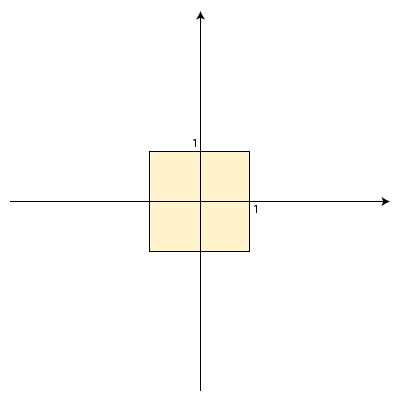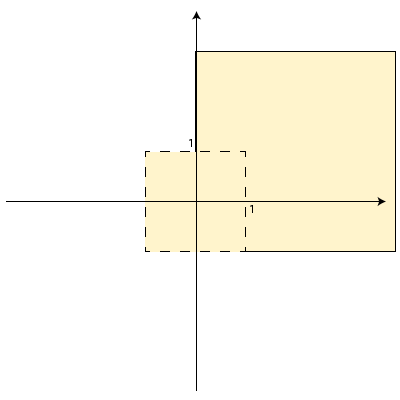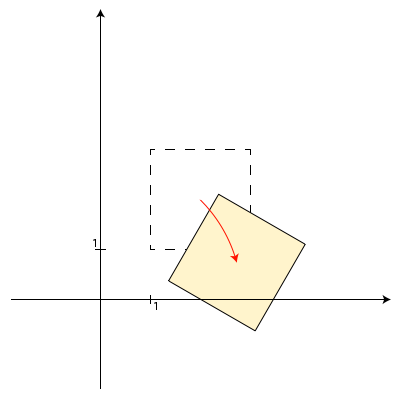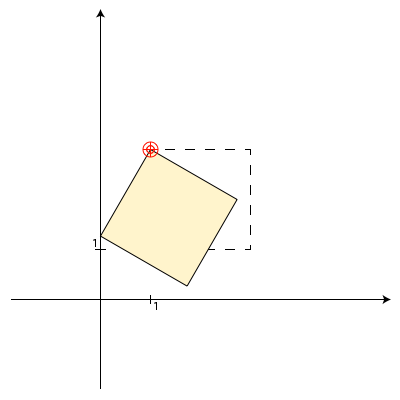# Understanding CSS3 2D Transforms

Klemen Slavič | March 15, 2011

## What Are Transform(ation)s?

Transformations, in their strictest and broadest mathematical sense, are mathematical operations that map a set of items X onto another set, Y. In geometric terms, this means that we can apply a mathematical operation on a set of objects in space to map them onto a new space geometry.

In layman's terms, we can take an object and apply what we call transformation groups. These are ordered lists of operations (called transforms) that we can use to movescalereflectrotate and skew objects. Sounds simple enough, right?

But before we jump right into the whole business of object manipulation, we need to look into how these transformation groups work, exactly. As mentioned, transformation groups are an ordered set of transformation operations, the implication being that the order of transformations matters and gives different results depending on the ordering of those operations. This is because transformations take into account every object's geometric properties, including its current position.

### Understanding the Transformation Origin

As an example, let's take a look at a very simple example. Say we have a rectangle of size 2×2 centered around the origin, (0,0):If we decide to scale the object by a factor of 2 and then move the object 1 unit to the right and 0.5 units upwards, we get the following result:The result is exactly what we expected – the square is now 4×4 units in size and its center moved to the point (1, 0.5). But what if we reversed the order of the operations? Let's find out:The result is correct, but it seems somewhat counter-intuitive – the center of the rectangle now lies at (2, 1) instead of (1, 0.5) as in the previous example. The reason behind this change in position is that transformations are dependent on the transformation origin and apply to all properties of the object, including its position. If the object is offset in relation to the transformation origin, its position gets transformed along with all the other properties. In our case, the x and y components of the object position were scaled by a factor of 2 as well.

If this seems a bit limiting or complicated, don't fret; although the transform origin is centered at (0,0) by default, we can change that by specifying it explicitly. Take the following example where we rotate a rectangle centered around (2,2) by 30 degrees clock-wise:Because the object was offset from the origin, the whole rectangle rotated around the origin. If we wanted to rotate the object around, say, its top left corner, we could move the transformation origin to that point and then rotate the object:There's a slight catch to this technique, though. You can only specify a single transformation group with a single transformation origin; this means that while you can specify an alternative origin, it cannot be changed while applying individual transformations within the group. The alternative is moving the object into position around the origin, applying the transformations and then moving the object back into place.

### Screen space vs. Euclidean space

The more astute among you might have probably noticed that I've used the classical Euclidean space in place of screen space to explain transformations. In reality, dealing with computer graphics (and, of course, web pages) entails a shift in the way the coordinate system is positioned.

As a convenience, the origin lies in the top left corner of every window and the y-axis points downwards so that every pixel on the screen can be described in terms of positive integers.

## TILT!

Having covered the mathematical aspects of transformations, let's take a look at how we can apply them to elements using CSS.

A word of caution before we proceed, though; since we're using features of CSS not yet present in every browser, we'll need to use vendor-spefic prefixes in addition to the standards-based CSS properties. See the Points of Interest section for ways of bypassing the redundancy of specifying cross-browser CSS transforms.

### Transformation origin

To specify the transformation origin, we use the `transform-origin` CSS property:

``````-moz-transform-origin: 50% 50%;
-webkit-transform-origin: 50% 50%;
-ms-transform-origin: 50% 50%;
-o-transform-origin: 50% 50%;
transform-origin: 50% 50%;
``````

By default the transform origin lies at the center of the object's bounding box and (0,0) denotes the top left corner of the object's bounding box.

### Transformation groups

To specify a transformation group, we use the `transform` CSS property and specify a list of transforms to apply, in order:

``````-moz-transform: <trans1> <trans2> ...;
-webkit-transform: <trans1> <trans2> ...;
-ms-transform: <trans1> <trans2> ...;
-o-transform: <trans1> <trans2> ...;
transform: <trans1> <trans2> ...;
``````

<trans1>, <trans2>, etc. in this case are different transforms, listed in white-space delimited format.

### Translation

To apply translation to an object, we use the `translate``translateX` and `translateY` keywords:

``````-moz-transform: translate(tx[, ty]) | translateX(tx) | translateY(ty);
-webkit-transform: translate(tx[, ty]) | translateX(tx) | translateY(ty);
-ms-transform: translate(tx[, ty]) | translateX(tx) | translateY(ty);
-o-transform: translate(tx[, ty]) | translateX(tx) | translateY(ty);
transform: translate(tx[, ty]) | translateX(tx) | translateY(ty);
``````

`tx` denotes a horizontal offset and `ty` vertical. The following example shows how to move an image 60 pixels to the right and 20 pixels down:

``````-moz-transform: translate(60px, 20px);
-webkit-transform: translate(60px, 20px);
-ms-transform: translate(60px, 20px);
-o-transform: translate(60px, 20px);
transform: translate(60px, 20px);
``````

### Scaling

To scale an object, we use the `scale``scaleX` and `scaleY` keywords:

``````-moz-transform: scale(sx[, sy]) | scaleX(sx) | scaleY(sy);
-webkit-transform: scale(sx[, sy]) | scaleX(sx) | scaleY(sy);
-ms-transform: scale(sx[, sy]) | scaleX(sx) | scaleY(sy);
-o-transform: scale(sx[, sy]) | scaleX(sx) | scaleY(sy);
transform: scale(sx[, sy]) | scaleX(sx) | scaleY(sy);
``````

Scaling scales the object by the factor specified as the argument. Each argument is a unitless number. If you use the `scale` keyword and only specify a single argument, it will perform a uniform scale (ie. will scale proportionally in both directions). Negative numbers will scale and mirror the object across the respective axis.

Here's an example of scaling down an object to 80% of its size towards to top right corner:

``````/* set the origin to the top right corner */
-moz-transform-origin: 100% 0;
-webkit-transform-origin: 100% 0;
-ms-transform-origin: 100% 0;
-o-transform-origin: 100% 0;
transform-origin: 100% 0;

/* scale it down to 80% */
-moz-transform: scale(0.8);
-webkit-transform: scale(0.8);
-ms-transform: scale(0.8);
-o-transform: scale(0.8);
transform: scale(0.8);
``````

If we wanted to mirror the image across the horizontal axis (vertical flip), we can use a negative`scaleY` value:

``````/* set the origin to the middle of the element (default) */
-moz-transform-origin: 50% 50%;
-webkit-transform-origin: 50% 50%;
-ms-transform-origin: 50% 50%;
-o-transform-origin: 50% 50%;
transform-origin: 50% 50%;

/* vertical flip across the middle of the image */
-moz-transform: scaleY(-1);
-webkit-transform: scaleY(-1);
-ms-transform: scaleY(-1);
-o-transform: scaleY(-1);
transform: scaleY(-1);
``````

### Rotation

To rotate an object, we use the `rotate` keyword:

``````-moz-transform: rotate(<angle>);
-webkit-transform: rotate(<angle>);
-ms-transform: rotate(<angle>);
-o-transform: rotate(<angle>);
transform: rotate(<angle>);
``````

<angle> denotes the angle by which to rotate the object around the transform origin and requires a unit (one of degrad or grad), a positive value meaning clockwise rotation. Example of rotating an object around its bottom right corner:

``````/* move the origin to the bottom right corner */
-moz-transform-origin: 100% 100%;
-webkit-transform-origin: 100% 100%;
-ms-transform-origin: 100% 100%;
-o-transform-origin: 100% 100%;
transform-origin: 100% 100%;

/* rotate 30 degrees clockwise */
-moz-transform: rotate(30deg);
-webkit-transform: rotate(30deg);
-ms-transform: rotate(30deg);
-o-transform: rotate(30deg);
transform: rotate(30deg);
``````

### Skewing

To skew an object, we use the `skew``skewX` and `skewY` keywords:

``````-moz-transform: skew(<angleX>[, <angleY>]) | skewX(<angleX>) | skewY(<angleY>);
-webkit-transform: skew(<angleX>[, <angleY>]) | skewX(<angleX>) | skewY(<angleY>);
-ms-transform: skew(<angleX>[, <angleY>]) | skewX(<angleX>) | skewY(<angleY>);
-o-transform: skew(<angleX>[, <angleY>]) | skewX(<angleX>) | skewY(<angleY>);
transform: skew(<angleX>[, <angleY>]) | skewX(<angleX>) | skewY(<angleY>);
``````

Since skewing only changes the orientation of the axes, it disregards the transformation origin. The angles specified use one of the following units: degrad and grad. The angles themselves work a bit counterintuitively, though; with rotation, a positive angle denotes a clockwise rotation, while skewing does the opposite. Also, while it may be logical to assume that `skewX` and `skewY` would affect the x and y axes respectively, they actually denote the direction in which the object is skewed.

Let's look at an example:

``````/* we fix the origin in the top left corner */
-moz-transform-origin: 0 0;
-webkit-transform-origin: 0 0;
-ms-transform-origin: 0 0;
-o-transform-origin: 0 0;
transform-origin: 0 0;

/* skew the object horizontally by 20 degress clockwise */
-moz-transform: skewX(-20deg);
-webkit-transform: skewX(-20deg);
-ms-transform: skewX(-20deg);
-o-transform: skewX(-20deg);
transform: skewX(-20deg);
``````

In this case, we see exactly how the transformation is applied. If using a negative argument with `skewX`, it will make the object lean to the right. Same goes for skewing vertically, but this time, a positive angle denotes a clockwise rotation:

``````/* we fix the origin in the top left corner */
-moz-transform-origin: 0 0;
-webkit-transform-origin: 0 0;
-ms-transform-origin: 0 0;
-o-transform-origin: 0 0;
transform-origin: 0 0;

/* skew the object vertically by 20 degress anti-clockwise */
-moz-transform: skewY(-20deg);
-webkit-transform: skewY(-20deg);
-ms-transform: skewY(-20deg);
-o-transform: skewY(-20deg);
transform: skewY(-20deg);
``````

While the way skewing works may be counterintuitive to most that approach this transformations, it does have a logical explanation: there's a rotation hidden in every skew operation.

What actually happens is that after an object is skewed (the respective axis being rotated), the browser automatically adds a rotation that realigns the chosen axis (negatively equal to the skewing angle), effectively rotating the other axis. So basically, you are rotating the x axis by the given amount when performing a `skewX` transformation, but it is immediately followed by a`rotate(-angle)` operation which realigns the x axis.

Still not sure which direction is which? Trial and error is usually the go-to method in this case. ;)

## Farewell Horizon, We Knew Thee Well

Now that we've mastered the individual transforms, let's look into the techniques of using transform groups to achieve certain effects.

A common technique to use with CSS transforms is the hover-tilt and hover-grow on buttons to communicate active elements and invite users to click on them. It is simply a composite transform that uses rotation and scaling to achieve the desired effect. Let's put a button in a box and apply the transforms:

``````button {
}

button:hover {
-moz-transform: rotate(3deg) scale(1.05);
-webkit-transform: rotate(3deg) scale(1.05);
-ms-transform: rotate(3deg) scale(1.05);
-o-transform: rotate(3deg) scale(1.05);
transform: rotate(3deg) scale(1.05);
}
``````

With a subtle rotation and scaling, we've created the desired effect – the button jumps out to the user, inviting them to click.

Transforms can even be combined with nested elements, each having their own set of transformation groups. A more elaborate example would be using a wrapping element around the button to rotate the wrapper in the opposite direction:

``````.wrapper {
margin: 5px;
background: red;
}

.wrapper:hover {
-moz-transform: rotate(-3deg) scale(1.05);
-webkit-transform: rotate(-3deg) scale(1.05);
-ms-transform: rotate(-3deg) scale(1.05);
-o-transform: rotate(-3deg) scale(1.05);
transform: rotate(-3deg) scale(1.05);
}

.wrapper button {
}

.wrapper button:hover {
-moz-transform: rotate(6deg) scale(1.05);
-webkit-transform: rotate(6deg) scale(1.05);
-ms-transform: rotate(6deg) scale(1.05);
-o-transform: rotate(6deg) scale(1.05);
transform: rotate(6deg) scale(1.05);
}
``````

In this case, hovering over the wrapper element will apply the wrapper transform to the element and everything it contains, but hovering over the button will transform the button according to its own transformation group. The two are independent, but hierarchical, since the parent transforms all its child elements.

### 2D transformations vs. 3D transformations

So at this point, you might be asking yourself what's stopping you from using a combination of these transforms to create a 3D transformation? Well, if you're content with using just orthographic projection, then you can use a combination of skewed objects to position and transform objects. If you're looking for true linear perspective (or any other non-linear projection), then you're out of luck.

You see, skewing only changes the angle between the two axes, while a third dimension coupled with perspective adds another dimension which affects the distance between two points when projected on a plane – in other words, the farther away you are from an object, the smaller it seems. That's something that cannot be accurately reproducted using only 2D transformations.

If you're interested in 3D transformations, there are currently two options available: true 3D transforms using a syntax similar the 2D transform CSS properties or WebGL. Neither are currently supported in major stable browsers, so usage is limited to the latest and greatest browsers, and also out of the scope of this article.

If you're looking for an introduction, you can read about it on the Webkit blog that explains the first implementation of the emerging 3D transforms.

## So, Where Can I Get Some?

Obviously, 2D transforms are something of a novelty among browsers; even though IE6 supported DXtransform through their proprietary filter CSS property, none of the other browsers implemented similar functionality until about 2 years ago, and even then it took a while for the other to pick up on it. The recent surge in browser development, though, caused all the major browser vendors to implement 2D transforms in their upcoming and current releases, some of them even using hardware acceleration to do the job.

Currently, the browsers supporting said transforms include the following flock:

Browser Since Implementing
Internet Explorer 5.5
9.0
• -ms-transform
Firefox (Gecko) 3.5 (1.9.1)
• -moz-transform
Opera 10.5
• -o-transform
Safari (WebKit) 3.1 (525)
• -webkit-transform
Chrome 1.0
• -webkit-transform (using WebKit engine)
iOS all versions -webkit-transform (using WebKit engine)

## Points of Interest

### Performance

With the browser support matrix you might be tempted to just go ahead and start sprinkling transforms left and right all over your sites. Technically, that is doable, but true cross-browser CSS transforms are costly, performance-wise.

For browsers that do not support hardware accelerated 2D transforms (practically every currently stable browser on Windows and Linux, for example), all of the transforms are performed on the CPU, with Firefox pre-4.0 and Internet Explorer pre-9 being the prime examples of slow rendering engines. While both execute transformations correctly, they do suffer a performance penalty, which is most noticeable on IE (this problem was addressed with IE9's direct DOM hardware rendering acceleration). Sites which contain many transforms will degrade performance and on IE might cause `z-index` stacking problems.

### Rendering

The other caveat to be aware of relates to Chrome on various platforms – the edges of rotated and skewed images (but not other DOM elements) tend to be jagged instead of antialiased on Windows. This problem is even worse on Linux, since any rotated text is not antialiased and parts of letters can appear disconnected and broken, rendering most small font sizes unreadable when transformed.

### Think of the childr… I mean, users!

If you're still set on using CSS transforms (and you should be!), you can still provide alternative presentations and graceful degradation for users that don't have 2D CSS transform capabilities using Modernizr. With it, you can detect features of the browser and use pure CSS to style alternative presentations or even hide elements that would otherwise appear out of order. The examples nested within `iframe`s in this article use it to present alternative presentations and notices to users whose browser does not support the W3C-like syntax of CSS transforms – try IE8-or an older version of, say, an order version of Camino or Konqueror.

Also, if you dislike having to write multiple versions of CSS attributes, you might want to consider using cssSandpaper which generate all the different vendor-specific and standard syntaxes so they work cross-browser. In addition, it also translates the W3C syntax into DirectX filters to make transforms work even with Internet Explorer 5.5!

## Have a go

That's the gist of 2D CSS transforms. Where we previously thought we were confined to the perpendicular geometry of the browser window, we can now slant, shear, stretch and squeeze text, images and even video! Think of all the bandwidth we can save on all the slanted text we've had to bitmap in the past.

Now go forth and nudge things about!